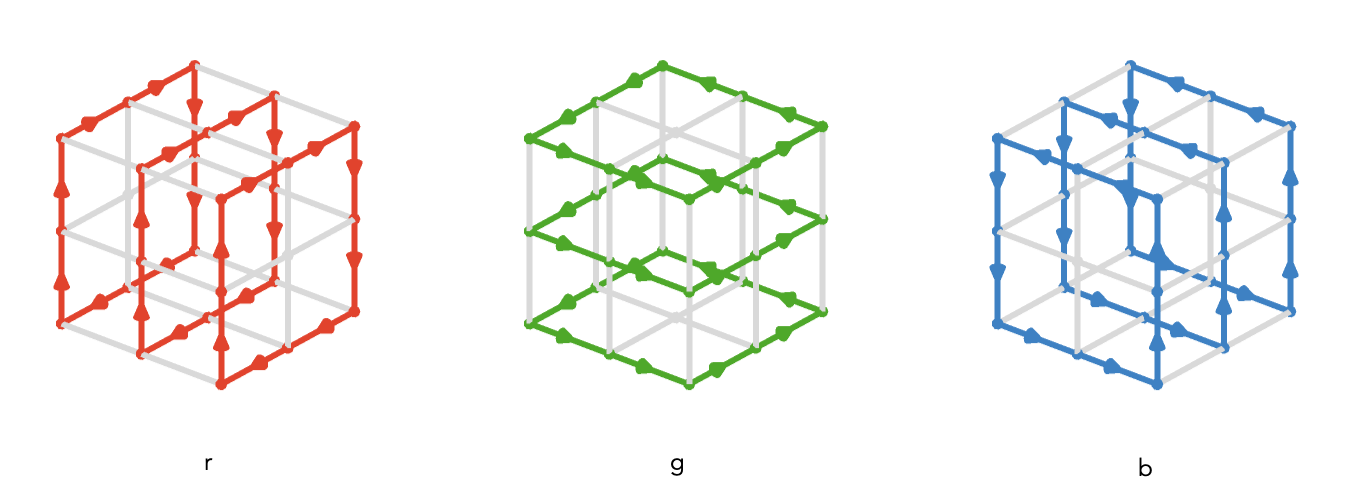$$\gdef\badDispatch#1{\textbf{\textcolor{e1432d}{#1}}} \gdef\noKatexForm#1{\badDispatch{#1}} \gdef\largeDot{\raisebox{0.06em}{\tiny∙}} \gdef\rarrbar{ {\raisebox{-0.05em}{→}\mkern{-0.13em}{\large\shortmid}}} \gdef\larrbar{ { {\large\shortmid}\mkern{-0.13em}{\raisebox{-0.05em}{←}}}} \gdef\suptrans{^\mathsf{T}} \gdef\supdagger{^\dagger} \gdef\rawsymbol#1{\operatorname{#1}} \gdef\colorsymbol#1#2{\textcolor{#1}{\rawsymbol{#2}}} \gdef\lsymbol#1{\colorsymbol{262626}{#1}} \gdef\msymbol#1{\colorsymbol{b50800}{#1}} \gdef\osymbol#1{\colorsymbol{00427f}{#1}} \gdef\lstring#1{\textcolor{666666}{\textrm{\textquotedblleft{#1}\textquotedblright}}} \gdef\boldForm#1{\textbf{#1}} \gdef\lrform#1{ {\textcolor{e1432d}{#1}}} \gdef\lgform#1{ {\textcolor{4ea82a}{#1}}} \gdef\lbform#1{ {\textcolor{3e81c3}{#1}}} \gdef\rform#1{ {\textcolor{e1432d}{#1}}} \gdef\gform#1{ {\textcolor{4ea82a}{#1}}} \gdef\bform#1{ {\textcolor{3e81c3}{#1}}} \gdef\rgform#1{ {\textcolor{dc841a}{#1}}} \gdef\gbform#1{ {\textcolor{47a5a7}{#1}}} \gdef\rbform#1{ {\textcolor{c74883}{#1}}} \gdef\waform#1{ {\textcolor{6b6b6b}{#1}}} \gdef\wbform#1{ {\textcolor{929292}{#1}}} \gdef\wcform#1{ {\textcolor{c5c5c5}{#1}}} \gdef\barToken{\mathbf{|}} \gdef\filledRectangleToken{▮} \gdef\emptyRectangleToken{▯} \gdef\filledSquareToken{■} \gdef\emptySquareToken{□} \gdef\filledToken{∙} \gdef\emptyToken{∘} \gdef\cardinalRewrite#1#2{\rewrite{#1}{#2}} \gdef\primed#1{ {#1}^{\prime}} \gdef\tinybullet{ {\tiny●}} \gdef\forwardFactor{\uparrow} \gdef\backwardFactor{\downarrow} \gdef\neutralFactor{\mid} \gdef\arrowhead{⏵} \gdef\deSymbol{ { { {\raisebox{1.1pt}{\tinybullet}\mkern{-1pt}{→}}}\,}} \gdef\ueSymbol{ {\raisebox{1pt}{\tinybullet\raisebox{-0.03em}{\mkern{-0.3pt}{\tiny──}\mkern{-0.3pt}}\tinybullet}\,}} \gdef\ldeSymbol{ { { {\raisebox{1.1pt}{\tinybullet}\mkern{-1pt}{\longrightarrow}}}\,}} \gdef\lueSymbol{ {\raisebox{1pt}{\tinybullet\raisebox{-0.03em}{\mkern{-0.3pt}{\tiny────}\mkern{-0.3pt}}\tinybullet}\,}} \gdef\de#1#2{ { {#1} \deSymbol {#2}}} \gdef\ue#1#2{ { {#1} \ueSymbol {#2}}} \gdef\tde#1#2#3{ { {#1} \overset{#3}{\deSymbol} {#2}}} \gdef\tue#1#2#3{ { {#1} \overset{#3}{\ueSymbol} {#2}}} \gdef\ltde#1#2#3{ { {#1} \overset{#3}{\ldeSymbol} {#2}}} \gdef\ltue#1#2#3{ { {#1} \overset{#3}{\lueSymbol} {#2}}} \gdef\mapsfrom{\htmlClass{hreflect}{\mapsto}} \gdef\longmapsfrom{\htmlClass{hreflect}{\longmapsto}} \gdef\diffd{𝕕} \gdef\partialdof#1{\partial {#1}} \gdef\emptySet{\empty} \gdef\unknown{\wbform{\text{?}}} \gdef\notApplicable{\wbform{\text{---}}} \gdef\textAnd{\text{\,and\,}} \gdef\identicallyEqualSymbol{\equiv} \gdef\congruentSymbol{\equiv} \gdef\isomorphicSymbol{\cong} \gdef\homotopicSymbol{\simeq} \gdef\approxEqualSymbol{\approx} \gdef\bijectiveSymbol{\cong} \gdef\defeq{\;≝\;} \gdef\defEqualSymbol{\;≝\;} \gdef\tailEqualSymbol{\underdot{=}} \gdef\headEqualSymbol{\dot{=}} \gdef\ruledelayed{:\to} \gdef\mathdollar{\text{\textdollar}} \gdef\hyphen{\text{-}} \gdef\endash{\text{--}} \gdef\emdash{\text{---}} \gdef\updownarrows{\uparrow\!\downarrow} \gdef\vthinspace{\mkern{1pt}} \gdef\dlq{\text{\textquotedblleft}} \gdef\drq{\text{\textquotedblright}} \gdef\dprime{ {\prime\prime}} \gdef\inverse#1{ { {#1}^{-1}}} \gdef\inverseSymbol{\inverse{□}} \gdef\groupDirectProduct#1{#1} \gdef\groupDirectProductSymbol{\times} \gdef\groupInverse{\inverse} \gdef\groupPower#1#2{ {#1}^{#2}} \gdef\groupCommutator#1#2{\left[{#1},{#2}\right]} \gdef\groupoidInverse{\inverse} \gdef\latticeBFS#1{\textrm{bfs}({#1})} \gdef\pathHomomorphism#1{#1} \gdef\groupoidFunction#1{#1} \gdef\groupoidHomomorphism#1{#1} \gdef\affineModifier#1{\overrightharpoon{#1}} \gdef\supercirc#1{#1^{\circ}} \gdef\supercircb#1{#1\!\degree} \gdef\toroidalModifier#1{\supercirc{#1}} \gdef\modulo#1{\supercirc{#1}} \gdef\dividesSymbol{\,|\,} \gdef\groupFunction#1{#1} \gdef\groupHomomorphism#1{#1} \gdef\automorphisms{\operatorname{Aut}} \gdef\endomorphisms{\operatorname{End}} \gdef\transportMap#1{\transportMapSymbol_{#1}} \gdef\transportMapSymbol{\tau} \gdef\action#1{#1} \gdef\selfAction#1{\hat{#1}} \gdef\actionGroupoid#1{\utilde{#1}} \gdef\cardinalGroup#1{G^*({#1})} \gdef\signed#1{ {#1}^*} \gdef\transportAtlas#1{T_{#1}} \gdef\inverted#1{\underline{#1}} \gdef\mirror#1{\overline{#1}} \gdef\pathComposeSymbol{ {\,∷\,}} \gdef\pathCompose#1#2{ {#1}\pathComposeSymbol{#2}} \gdef\translateSymbol{\uparrow} \gdef\backwardTranslateSymbol{\downarrow} \gdef\pathTranslate#1#2{ {#1}\,\translateSymbol\,{#2}} \gdef\pathLeftTranslate#1{ {#1}{\,\translateSymbol}} \gdef\pathBackwardTranslate#1#2{ {#1}{\,\backwardTranslateSymbol\,}{#2}} \gdef\pathLeftBackwardTranslate#1{ {#1}{\,\backwardTranslateSymbol}} \gdef\compactCovariantDifference#1#2{\Delta_{#1}\,{#2}} \gdef\covariantDifference{ {#1}\,\Delta\,{#2}} \gdef\forwardDifference{\Delta^{+}} \gdef\backwardDifference{\Delta^{-}} \gdef\centralDifference{\Delta} \gdef\pathForwardDifference#1{\forwardDifference_{#1}} \gdef\pathBackwardDifference#1{\backwardDifference_{#1}} \gdef\pathCentralDifference#1{\centralDifference_{#1}} \gdef\pathHead#1{\pathHeadVector{#1}} \gdef\pathTail#1{\pathTailVector{#1}} \gdef\pathHeadVector#1{ {#1}^{\bullet}} \gdef\pathTailVector#1{ {#1}_{\bullet}} \gdef\pathReverse#1{ {#1}^{\dagger}} \gdef\pathIntegral#1#2{ {#1} \int {#2}} \gdef\pathIntegralSymbol{ {\int}} \gdef\pathDot#1#2{ {#1} \cdot {#2}} \gdef\pathDotSymbol{ {\cdot}} \gdef\compactBasis#1{\mathscr{B}} \gdef\length{\operatorname{len}} \gdef\signedLength{\operatorname{len^*}} \gdef\andFn{\operatorname{and}} \gdef\orFn{\operatorname{or}} \gdef\notFn{\operatorname{not}} \gdef\vertexList{\operatorname{vertices}} \gdef\vertexList{\operatorname{vertices}} \gdef\edgeList{\operatorname{edges}} \gdef\pathList{\operatorname{paths}} \gdef\cardinalList{\operatorname{cards}} \gdef\signedCardinalList{\operatorname{cards^*}} \gdef\wordOf{\operatorname{word}} \gdef\headVertex{\operatorname{head}} \gdef\tailVertex{\operatorname{tail}} \gdef\basis{\operatorname{basis}} \gdef\split{\operatorname{split}} \gdef\lcm{\operatorname{lcm}} \gdef\minimalContractionSets{\operatorname{MCSets}} \gdef\minimalContractions{\operatorname{MC}} \gdef\grade{\operatorname{grade}} \gdef\support{\operatorname{support}} \gdef\coefficient{\operatorname{coeff}} \gdef\domain{\operatorname{domain}} \gdef\codomain{\operatorname{codomain}} \gdef\modFunction{\operatorname{mod}} \gdef\isPrime#1{#1\textrm{ prime}} \gdef\blank{\_} \gdef\emptyWord{} \gdef\multiwordSymbol#1{\mathbf{#1}} \gdef\wordSymbol#1{\mathtt{#1}} \gdef\word#1{#1} \gdef\pathMap#1{#1} \gdef\function#1{#1} \gdef\imageModifier#1{ {#1}^{\rightarrow}} \gdef\preimageModifier#1{ {#1}^{\leftarrow}} \gdef\multiImageModifier#1{ {#1}^{\Rightarrow}} \gdef\multiPreimageModifier#1{ {#1}^{\Leftarrow}} \gdef\functionComposition#1{#1} \gdef\functionCompositionSymbol{∘} \gdef\route#1#2#3{[{#1}\!:\!{#2}\!:\!{#3}]} \gdef\multiroute#1#2#3{[{#1}\!:\!{#2}\!:\!{#3}]} \gdef\pathWord#1#2#3{ {#1}\!:\!{#2}\!:\!{#3}} \gdef\nullPath{\bot} \gdef\nullElement{\bot} \gdef\path#1{#1} \gdef\vert#1{#1} \gdef\underdot#1{\underset{\raisebox{0.3em}{.}}{#1}} \gdef\tvert#1{\underdot{#1}} \gdef\hvert#1{\dot{#1}} \gdef\edge#1{#1} \gdef\card#1{\mathtt{#1}} \gdef\path#1{#1} \gdef\quiver#1{#1} \gdef\bindingRuleSymbol{\to} \gdef\compactBindingRuleSymbol{:} \gdef\cayleyQuiverSymbol#1{\selfAction{#1}} \gdef\bindCayleyQuiver#1#2{\selfAction{#1}[#2]} \gdef\bindActionQuiver#1#2{#1[#2]} \gdef\bindSize#1#2{#1(#2)} \gdef\bindCardSize#1#2{#1[#2]} \gdef\bindCards#1#2{#1[#2]} \gdef\subSize#1#2{#1_{#2}} \gdef\gridQuiver#1{\textrm{Grid}^{#1}} \gdef\treeQuiver#1{\textrm{Tree}^{#1}} \gdef\bouquetQuiver#1{\textrm{Bq}^{#1}} \gdef\lineQuiver{\textrm{L}} \gdef\cycleQuiver{\textrm{C}} \gdef\squareQuiver{\textrm{Sq}} \gdef\cubicQuiver{\textrm{Cbc}} \gdef\triangularQuiver{\textrm{Tri}} \gdef\hexagonalQuiver{\textrm{Hex}} \gdef\rhombilleQuiver{\textrm{Rmb}} \gdef\limit#1#2{\lim_{#2}\,#1} \gdef\realVectorSpace#1{\mathbb{R}^{#1}} \gdef\realVectorSpace#1{\mathbb{C}^{#1}} \gdef\matrixRing#1#2{M_{#2}(#1)} \gdef\groupoid#1{#1} \gdef\group#1{#1} \gdef\field#1{#1} \gdef\ring#1{#1} \gdef\indeterminate#1{#1} \gdef\semiring#1{#1} \gdef\sym#1{#1} \gdef\matrix#1{#1} \gdef\polynomial#1{#1} \gdef\polynomialRing#1#2{#1[{#2}]} \gdef\routeSymbol#1{#1} \gdef\multirouteSymbol#1{\mathbf{#1}} \gdef\planSymbol#1{\mathbf{#1}} \gdef\ringElement#1{#1} \gdef\matrixPart#1#2#3{#1\llbracket{#2,#3}\rrbracket} \gdef\matrixRowPart#1{#1} \gdef\matrixColumnPart#1{#1} \gdef\subMatrixPart#1#2#3{#1_{#2,#3}} \gdef\matrixDotSymbol{\cdot} \gdef\matrixPlusSymbol{+} \gdef\wordGroup#1{\wordGroupSymbol_{#1}} \gdef\wordGroupSymbol{\Omega} \gdef\wordRing#1{\wordRingSymbol_{#1}} \gdef\wordRingSymbol{\Omega\!\degree} \gdef\linearCombinationCoefficient#1{ {\textcolor{888888}{#1}}} \gdef\plan#1{#1} \gdef\planRing#1{\planRingSymbol_{#1}} \gdef\planRingSymbol{\Phi} \gdef\basisPath#1#2{\mathbf{#1}_{#2}} \gdef\basisPathWeight#1#2{ {#1}_{#2}} \gdef\unitSymbol{\mathbf{e}} \gdef\unitVertexField{\unitSymbol_1} \gdef\forwardSymbol{f} \gdef\backwardSymbol{b} \gdef\symmetricSymbol{s} \gdef\antisymmetricSymbol{a} \gdef\wordVector#1#2{\unitSymbol_{#1}^{#2}} \gdef\gradOf#1{\grad\,{#1}} \gdef\grad{\nabla} \gdef\divOf#1{\div\,{#1}} \gdef\div{\dot{\nabla}} \gdef\laplacianOf#1{\laplacian\,{#1}} \gdef\laplacian{\ddot{\nabla}} \gdef\suchThat#1#2{ {#1}|{#2}} \gdef\chart#1{\chartSymbol_{#1}} \gdef\chartSymbol{C} \gdef\graphRegionIntersectionSymbol{\cap} \gdef\graphRegionUnionSymbol{\cup} \gdef\pathIso{\simeq} \gdef\factorial#1{#1!} \gdef\power#1#2{ {#1}^{#2}} \gdef\repeatedPower#1#2{ {#1}^{#2}} \gdef\contractionLattice#1{\operatorname{Con}(#1)} \gdef\contractedRelation#1{\sim_{#1}} \gdef\isContracted#1#2{ {#1} \sim {#2}} \gdef\isContractedIn#1#2#3{ {#1} \sim_{#3} {#2}} \gdef\isNotContracted#1#2{ {#1} \not \sim {#2}} \gdef\isNotContractedIn#1#2#3{ {#1} \not \sim_{#3} {#2}} \gdef\contractionSum#1{#1} \gdef\contractionSumSymbol{\sqcup} \gdef\contractionProduct#1{#1} \gdef\contractionProductSymbol{ {\cdot}} \gdef\graph#1{#1} \gdef\graphHomomorphism#1{#1} \gdef\coversSymbol{\sqsupseteq} \gdef\coveredBySymbol{\sqsubseteq} \gdef\strictlyCoversSymbol{\sqsupset} \gdef\strictlyCoveredBySymbol{\sqsubset} \gdef\covering#1#2#3{ {#2} \sqsupseteq_{#1} {#3}} \gdef\powerSet#1{\powerSetSymbol({#1})} \gdef\powerSetSymbol{\mathscr{P}} \gdef\existsForm#1#2{\exists\,{#1}\,:\,{#2}} \gdef\forAllForm#1#2{\forall\,{#1}\,:\,{#2}} \gdef\notted#1{\notSymbol {#1}} \gdef\andSymbol{\land} \gdef\orSymbol{\lor} \gdef\notSymbol{\lnot} \gdef\latticeElement#1{#1} \gdef\latticeMeetSymbol{\wedge} \gdef\latticeJoinSymbol{\vee} \gdef\latticeTop{\top} \gdef\latticeBottom{\bot} \gdef\latticeGreaterSymbol{>} \gdef\latticeGreaterEqualSymbol{\ge} \gdef\latticeLessSymbol{<} \gdef\latticeLessEqualSymbol{\le} \gdef\grpname#1{\operatorname{\mathsf{#1}}} \gdef\mkg#1#2#3{\grpname{#1}({#2},{#3})} \gdef\mka#1#2#3{\mathfrak{#1}({#2},{#3})} \gdef\generalLinearAlgebra#1#2{\mka{gl}{#1}{#2}} \gdef\generalLinearGroup#1#2{\mkg{GL}{#1}{#2}} \gdef\specialLinearAlgebra#1#2{\mka{sl}{#1}{#2}} \gdef\specialLinearGroup#1#2{\mkg{SL}{#1}{#2}} \gdef\projectiveGeneralLinearAlgebra#1#2{\mka{pgl}{#1}{#2}} \gdef\projectiveGeneralLinearGroup#1#2{\mkg{PGL}{#1}{#2}} \gdef\projectiveSpecialLinearAlgebra#1#2{\mka{psl}{#1}{#2}} \gdef\projectiveSpecialLinearGroup#1#2{\mkg{PSL}{#1}{#2}} \gdef\orthogonalAlgebra#1#2{\mka{o}{#1}{#2}} \gdef\orthogonalGroup#1#2{\mkg{O}{#1}{#2}} \gdef\specialOrthogonalAlgebra#1#2{\mka{so}{#1}{#2}} \gdef\specialOrthogonalGroup#1#2{\mkg{SO}{#1}{#2}} \gdef\unitaryAlgebra#1{\mka{u}{#1}{#2}} \gdef\unitaryGroup#1{\mkg{U}{#1}{#2}} \gdef\specialUnitaryAlgebra#1#2{\mka{su}{#1}{#2}} \gdef\specialUnitaryGroup#1#2{\mkg{su}{#1}{#2}} \gdef\spinAlgebra#1#2{\mka{spin}{#1}{#2}} \gdef\spinGroup#1#2{\mkg{Spin}{#1}{#2}} \gdef\pinAlgebra#1#2{\mka{pin}{#1}{#2}} \gdef\pinGroup#1#2{\mkg{Pin}{#1}{#2}} \gdef\symmetricGroup#1{\grpname{Sym}({#1})} \gdef\presentation#1{#1} \gdef\groupPresentation#1#2{\left\langle\,{#1}\,\,\middle|\mathstrut\,\,{#2}\,\right\rangle} \gdef\groupRelationIso{=} \gdef\groupGenerator#1{#1} \gdef\groupRelator#1{#1} \gdef\groupElement#1{#1} \gdef\groupoidElement#1{#1} \gdef\iconstruct#1#2{ {#1}\,\,\middle|{\large\mathstrut}\,\,{#2}} \gdef\setConstructor#1#2{\left\{\,\iconstruct{#1}{#2}\,\right\}} \gdef\multisetConstructor#1#2{\left\{\mkern{-2.3pt}\left|\,\,\iconstruct{#1}{#2}\,\right|\mkern{-2.3pt}\right\}} \gdef\multisets#1{\mathscr{M}({#1})} \gdef\signedMultisets#1{\mathscr{M^*}({#1})} \gdef\setSymbol#1{#1} \gdef\multisetSymbol#1{#1} \gdef\setElementSymbol#1{#1} \gdef\multisetElementSymbol#1{#1} \gdef\multisetMultiplicitySymbol{\raisebox{.1em}{\small\#}\mkern{.1em}} \gdef\boundMultiplicityFunction#1{\operatorname{ {#1}^{\sharp}}} \gdef\constructor#1#2{\left.{#1}\,\,\middle|\mathstrut\,\,{#2}\right.} \gdef\elemOf#1#2{ { {#1} \in {#2} }} \gdef\notElemOf#1#2{ { {#1} \notin {#2} }} \gdef\edgeOf#1#2{ { {#1} {\in}_E {#2} }} \gdef\vertOf#1#2{ { {#1} {\in}_V {#2} }} \gdef\pathOf#1#2{ { {#1} {\in}_P {#2} }} \gdef\pathType#1{\operatorName{path}{#1}} \gdef\vertexType#1{\operatorName{vertex}{#1}} \gdef\edgeType#1{\operatorName{edge}{#1}} \gdef\multisetType#1{\operatorName{mset}{#1}} \gdef\signedMultisetType#1{\operatorName{mset^*}{#1}} \gdef\fromTo#1{#1} \gdef\fromToSymbol{\mapsto} \gdef\vertexCountOf#1{|{#1}|} \gdef\vertices#1{ V_{#1} } \gdef\edges#1{ E_{#1} } \gdef\vertexField#1{#1} \gdef\edgeField#1{#1} \gdef\pathVector#1{\mathbf{#1}} \gdef\pathVectorSpace#1{\mathscr{#1}} \gdef\baseField#1{#1} \gdef\finiteField#1{\mathbb{F}_{#1}} \gdef\functionSpace#1#2{#2^{#1}} \gdef\pathGroupoid#1{ { \Gamma_{#1} }} \gdef\forwardPathQuiver#1#2{ {\overrightharpoon{#1}_{#2}}} \gdef\backwardPathQuiver#1#2{ {\overrightharpoon{#1}^{#2}}} \gdef\pathQuiver#1{ {\overrightharpoon{#1}}} \gdef\mto#1#2{ {#1}\mapsto{#2}} \gdef\mtoSymbol{\mapsto} \gdef\groupWordRewriting#1{\langle{#1}\rangle} \gdef\rewrite#1#2{ {#1}\mapsto{#2}} \gdef\rewritingRule#1#2{ {#1}\mapsto{#2}} \gdef\rewritingSystem#1{\mathcal{#1}} \gdef\multiwayBFS#1{\textrm{bfs}({#1})} \gdef\rewritingStateBinding#1#2{ {\left.{#1}\!\mid\!{#2}\right.}} \gdef\rewritingRuleBinding#1#2{#1{\left[\,{#2}\,\right]}} \gdef\namedSystem#1{\mathsf{#1}} \gdef\genericRewritingSystem{\namedSystem{System}} \gdef\stringRewritingSystem{\namedSystem{Str}} \gdef\turingMachineRewritingSystem{\namedSystem{TM}} \gdef\cellularAutomatonRewritingSystem{\namedSystem{CA}} \gdef\graphRewritingSystem{\namedSystem{Gr}} \gdef\hypergraphRewritingSystem{\namedSystem{HGr}} \gdef\petriNetRewritingSystem{\namedSystem{Petri}} \gdef\ncard#1{\card{\inverted{#1}}} \gdef\mcard#1{\card{\mirror{#1}}} \gdef\nmcard#1{\card{\inverted{\mirror{#1}}}} \gdef\assocArray#1{\left\langle {#1} \right\rangle} \gdef\set#1{\{ {#1} \}} \gdef\list#1{\{ {#1} \}} \gdef\multiset#1{\lBrace {#1} \rBrace} \gdef\permutationCycle#1{#1} \gdef\permutationCycleSymbol{\to} \gdef\permutationSet#1{#1} \gdef\permutationSetSymbol{;} \gdef\transposition#1#2{ {#1} \leftrightarrow {#2}} \gdef\tuple#1{( {#1} )} \gdef\concat#1{ {#1} } \gdef\paren#1{\left( {#1} \right)} \gdef\ceiling#1{\lceil{#1}\rceil} \gdef\floor#1{\lfloor{#1}\rfloor} \gdef\translationVector#1{\left[{#1}\right]_{\textrm{T}}} \gdef\quotient#1#2{ {#1} / {#2}} \gdef\compactQuotient#1#2#3{ {#1}\;{\upharpoonright_{#2}}\;{#3}} \gdef\multilineQuotient#1#2{\frac{#1}{#2}} \gdef\idElem#1{e_{#1}} \gdef\minus#1{-{#1}} \gdef\elem{\ \in\ } \gdef\vsp{\mkern{0.4pt}} \gdef\iGmult{\vsp} \gdef\gmult{\vsp\ast\vsp} \gdef\Gmult{\vsp\ast\vsp} \gdef\gdot{\vsp\cdot\vsp} \gdef\gDot{\vsp{\,\largeDot\,}\vsp} \gdef\ellipsis{\dots} \gdef\verticalEllipsis{\vdots} \gdef\appliedRelation#1{#1} \gdef\setUnionSymbol{\cup} \gdef\setIntersectionSymbol{\cap} \gdef\setRelativeComplementSymbol{\backslash} \gdef\multisetUnionSymbol{\cup} \gdef\multisetIntersectionSymbol{\cap} \gdef\multisetRelativeComplementSymbol{\backslash} \gdef\multisetSumSymbol{+} \gdef\cartesianProductSymbol{\times} \gdef\functionSignature#1#2#3{ { {#1} : {#2} \to {#3}}} \gdef\poly#1{#1} \gdef\quiverProdPoly#1{#1} \gdef\quiverProdPower#1#2{#1^{#2}} \gdef\quiverProdTimes#1{#1} \gdef\parenLabeled#1#2{#1\ ({#2})} \gdef\parenRepeated#1#2{\parenLabeled{#1}{ {#2}\text{ times}}} \gdef\underLabeled#1#2{\underbrace{#1}_{#2}} \gdef\underRepeated#1#2{\underbrace{#1}_{#2\text{ times}}} \gdef\overLabeled#1#2{\overbrace{#1}^{#2}} \gdef\overRepeated#1#2{\overbrace{#1}^{#2\text{ times}}} \gdef\modLabeled#1#2{ {#1 }\textrm{ mod }{#2}} \gdef\freeGroup#1{F_{#1}} \gdef\cyclicGroup#1{\mathbb{Z}_{#1}} \gdef\componentSuperQuiverOfSymbol{\succ} \gdef\setCardinality#1{|{#1}|} \gdef\dependentQuiverProductSymbol{\,\times\,} \gdef\independentQuiverProductSymbol{\,⨝\,} \gdef\graphUnionSymbol{\sqcup} \gdef\graphProductSymbol{\times} \gdef\inlineProdSymbol{\mid} \gdef\serialCardSymbol{ {:}} \gdef\parallelCardSymbol{ {\mid}} \gdef\cardinalSequenceSymbol{ {:}} \gdef\cardinalProductSymbol{\inlineProdSymbol} \gdef\vertexProductSymbol{\!\inlineProdSymbol\!} \gdef\edgeProductSymbol{\inlineProdSymbol} \gdef\indexSum#1#2#3{ {\sum_{#2}^{#3} #1}} \gdef\indexProd#1#2#3{ {\prod_{#2}^{#3} #1}} \gdef\indexMax#1#2#3{ {\max_{#2}^{#3} #1}} \gdef\indexMin#1#2#3{ {\min_{#2}^{#3} #1}} \gdef\oneTo#1{1..{#1}} \gdef\zeroTo#1{0..{#1}} \gdef\qstring#1{\mathtt{"}{#1}\mathtt{"}} \gdef\qchar#1{\mathtt{'}{#1}\mathtt{'}} \gdef\lstr#1{\mathtt{#1}} \gdef\lchar#1{\mathtt{#1}} \gdef\string#1{ {#1}} \gdef\character#1{ {#1}} \gdef\homomorphismMapping#1{\assocArray{#1}} \gdef\translationPresentation#1{\textrm{Z}_{#1}} \gdef\starTranslationPresentation#1{\textrm{Z}^*_{#1}} \gdef\translationPathValuation#1{\mathcal{\overrightharpoon Z}_{#1}} \gdef\starTranslationPathValuation#1{\overrightharpoon{\mathcal{Z}^*_{#1}}} \gdef\translationWordHomomorphism#1{\mathcal{Z}_{#1}} \gdef\starTranslationWordHomomorphism#1{\mathcal{Z}^*_{#1}} \gdef\translationCardinalValuation#1{\textrm{T}_{#1}} \gdef\starTranslationCardinalValuation#1{\textrm{T}^*_{#1}}$$

In Graphs and quivers we introduce the notion of a cardinal quiver and describe the local uniqueness property. We also enumerate some small quivers.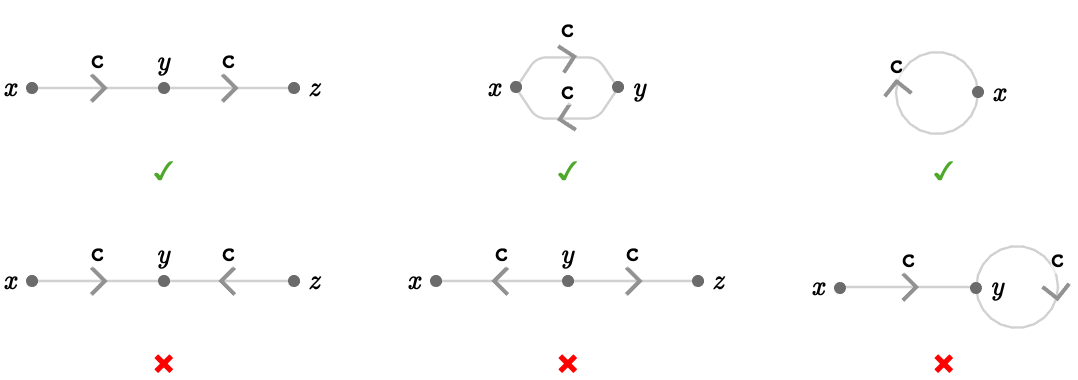In Transitive quivers we introduce families of simple transitive quivers: the line, square, triangular, cubic, grid, bouquet, and tree quivers.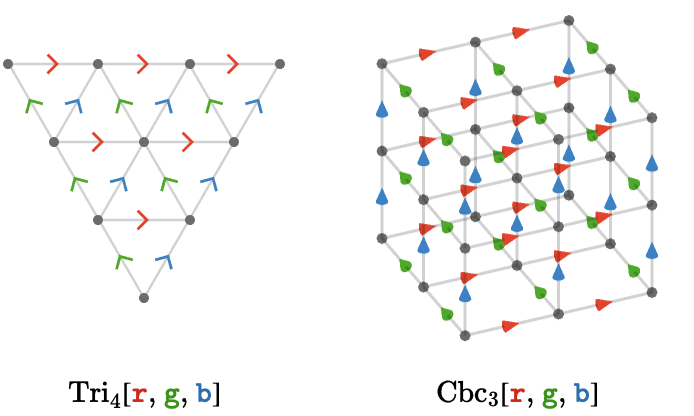In Path groupoids we define paths on a quiver, consider their path words, and show the paths form a path groupoid.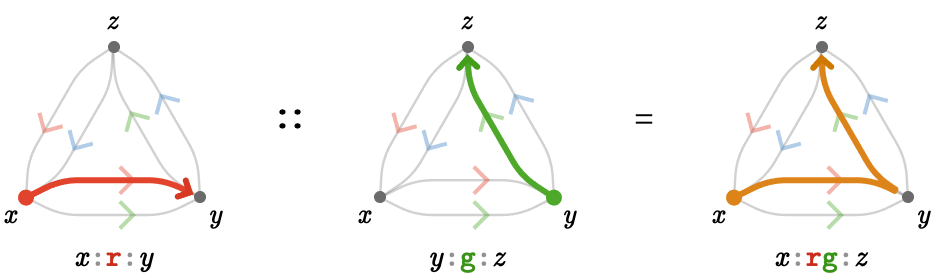In Word groups we define the word group, which describes how we can build path words out of individual cardinals.

In Path homomorphisms we define path homomorphisms between quivers, list some properties, and examine some examples of path homomorphisms to build intuition. We also consider affine path homomorphisms and automorphisms of quivers.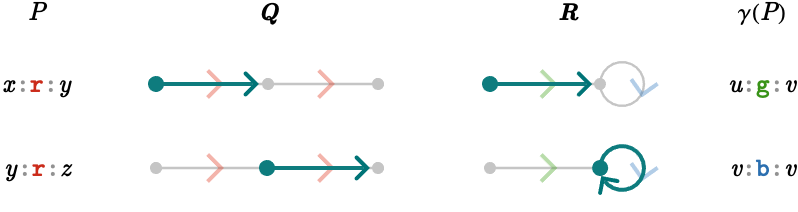In Multisets we set the stage for later developments by defining the useful datastructure known as the multiset. We explain how to form sums, unions, and intersections of multisets, and explain the behavior of images of functions on multisets. We generalize the multiset to the signed multiset.

In Word rings we define the word ring, which is the group ring of the word group. The word ring models linear combinations of words. We show how to interpret elements of the word ring as modelling multiwords: signed multisets of words.

In Adjacency we contemplate how to extend the concept of an adjacency matrix to model the cardinal structure of a quiver. We do this by defining the plan ring: a matrix ring over the word ring. Under multiset duality, matrices in this ring are dual to plans, which are multisets of (hypothetical) paths.

In Cayley quivers we define how groups and their presentations give rise to Cayley quivers and show how the simple quivers we examined in Transitive quivers are thus generated as presentations of $$\group{\power{\group{\mathbb{Z}}}{\sym{n}}}$$.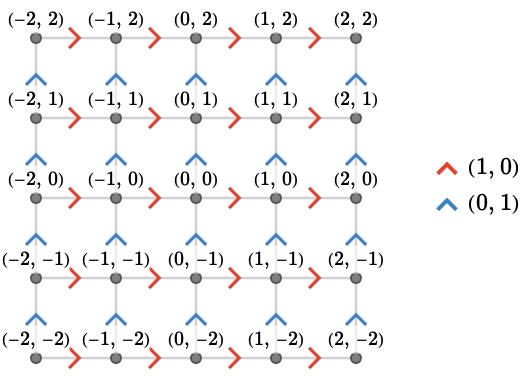In Action groupoids we recall group actions and understand Cayley quivers as instances of action quivers associated with actions of groups on themselves.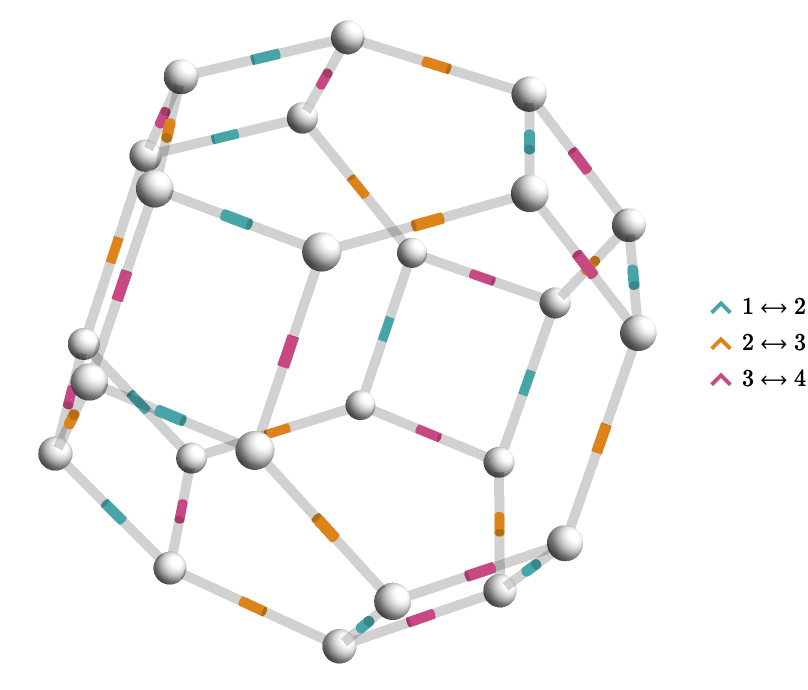In Path quivers we define the forward, backward, and affine path quiver, which describe the possible paths of a quiver using another quiver.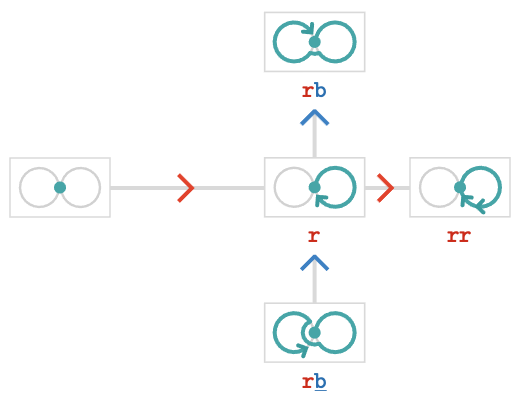In Path quotients we define path valuations, and use these to construct quotients of path quivers.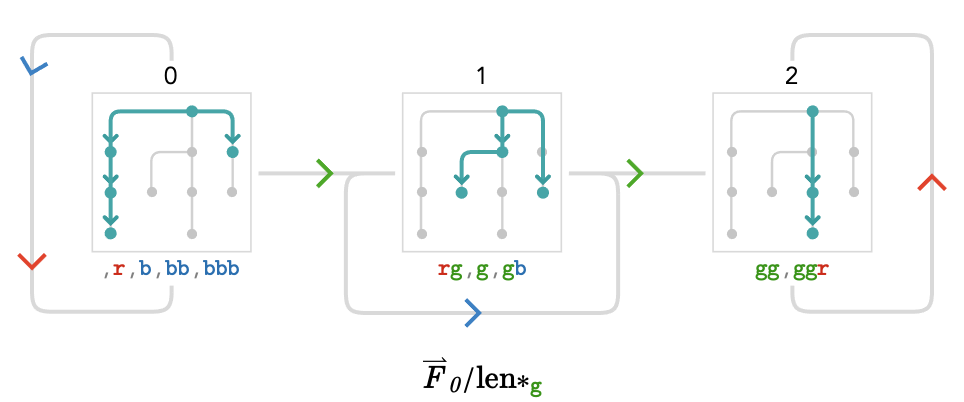In Lattice quivers we generate some of the familiar transitive quivers as the quotients of one-vertex fundamental quivers by affine path valuations associated with group presentations of $$\group{\power{\group{\mathbb{Z}}}{\sym{n}}}$$. We explain how such a quotient can be seen both as a breadth-first-search and as a Cayley quiver.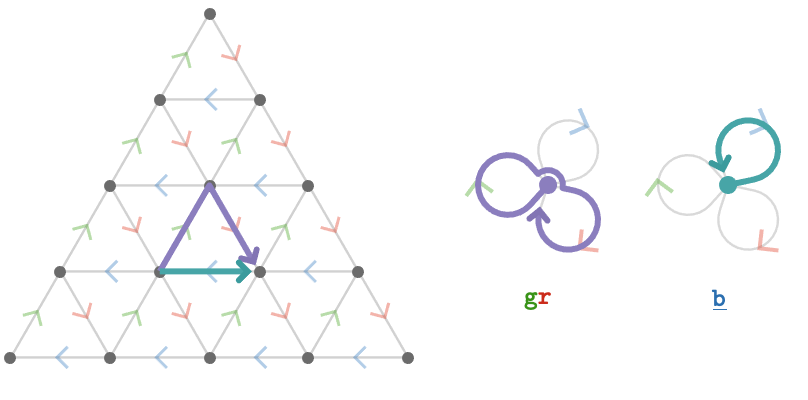In Intransitive lattices, we use quotients of fundamental quivers with two or more vertices to generate intransitive quivers such as the hexagonal and rhombille quivers.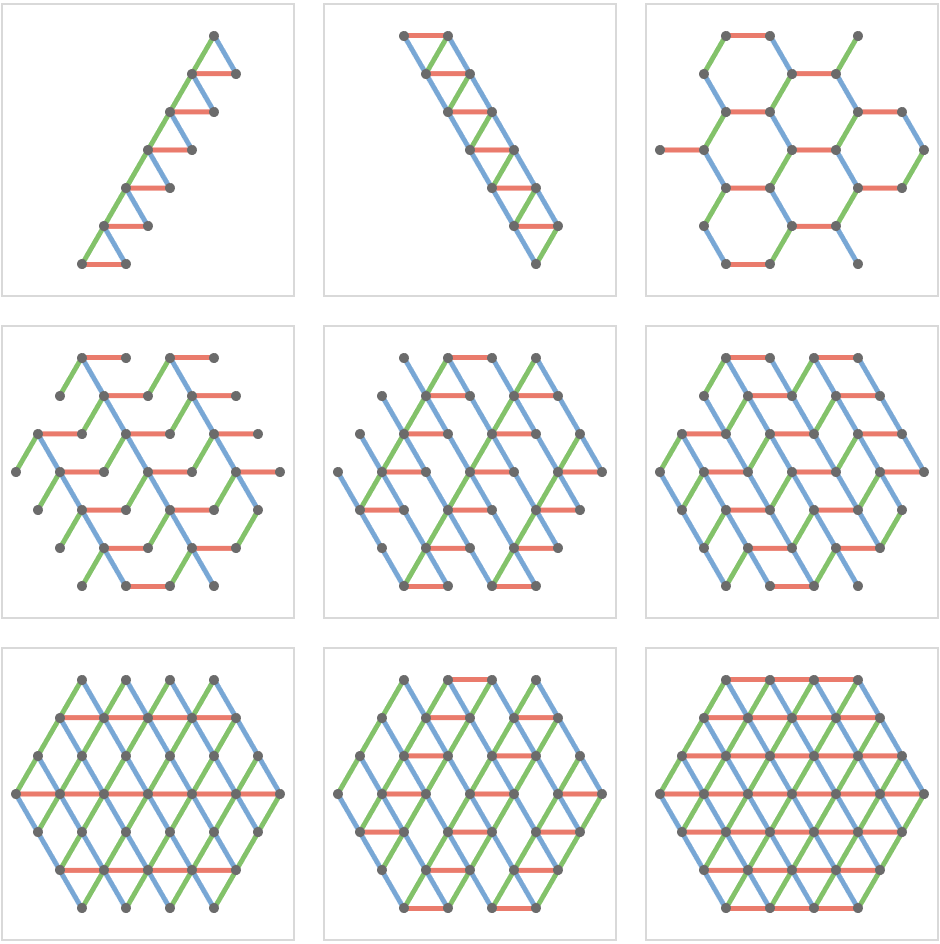In Toroidal lattices, we generate square, triangular, and hexagonal toroidal quivers as the Cayley quivers of presentations of finite Abelian groups, and plot these in three dimensions and on the "modular plane".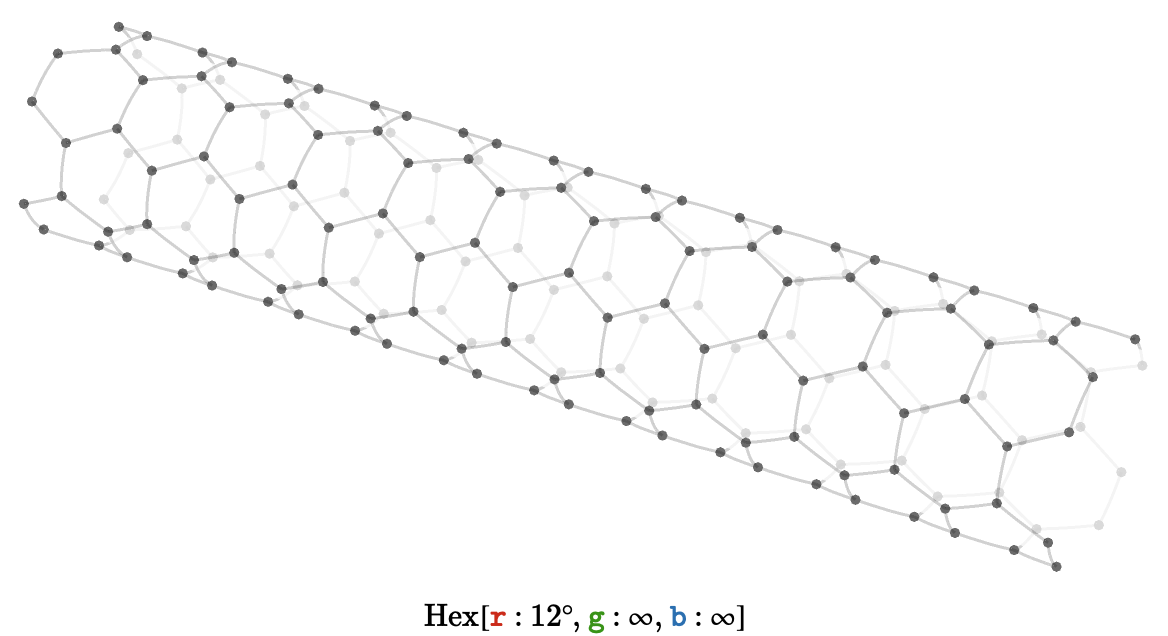In Noncommutativity, we consider quivers obtained as the Cayley quivers of non-Abelian groups.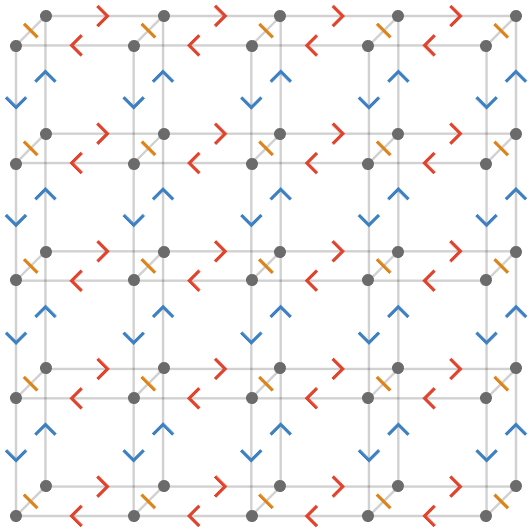In Rewriting systems, we define rewriting systems and the quivers they generate.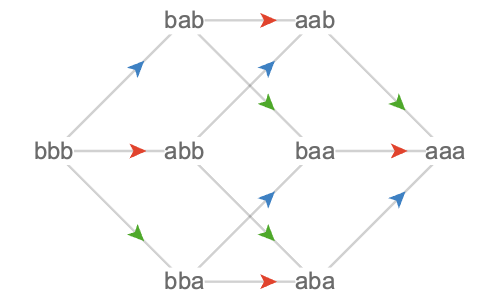In Coverings, we define graph and quiver coverings, and explain their connection to path homomorphisms.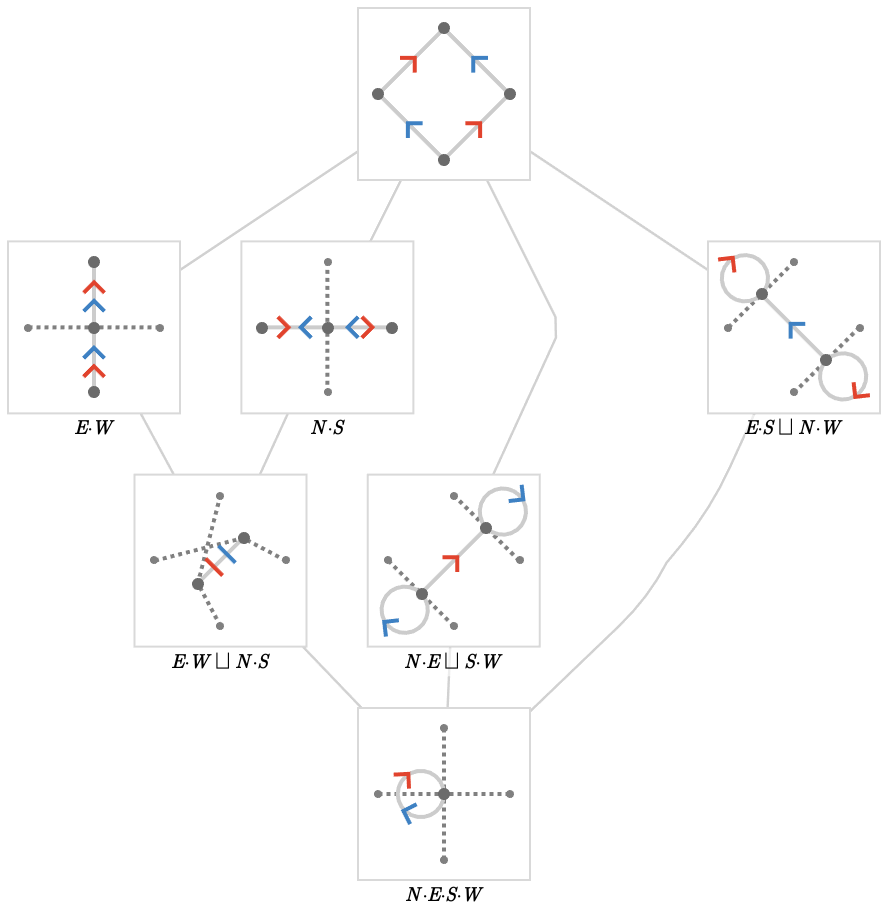In Contraction lattices, we examine coverings derived from contractions of vertices, and show possess the structure of an order-theoretic lattice.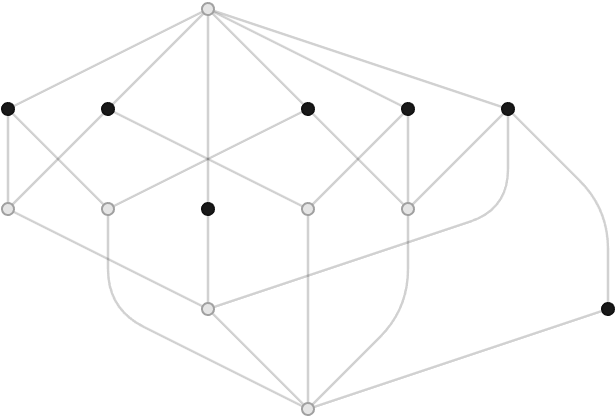In Vertex colorings, we enumerate some regular vertex colorings of transitive quivers, and explain their connection to contraction lattices.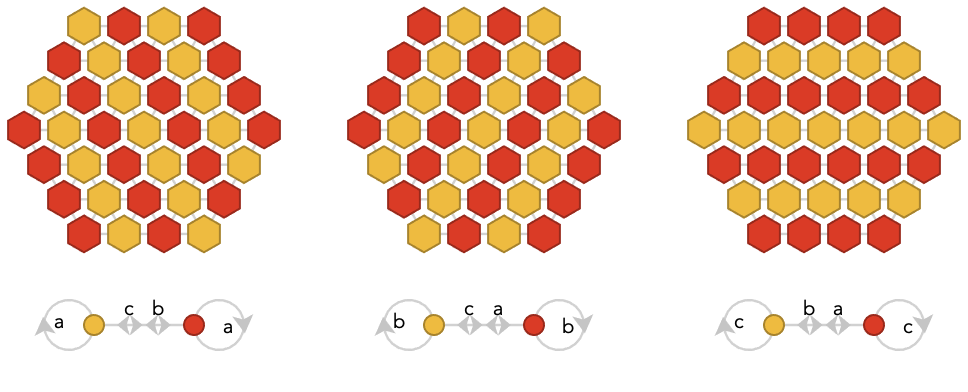In Quiver products, we generalize from the depedendent, independent, and Cartesian products of quivers to obtain a general method for multiplying quivers together using arrow polynomials.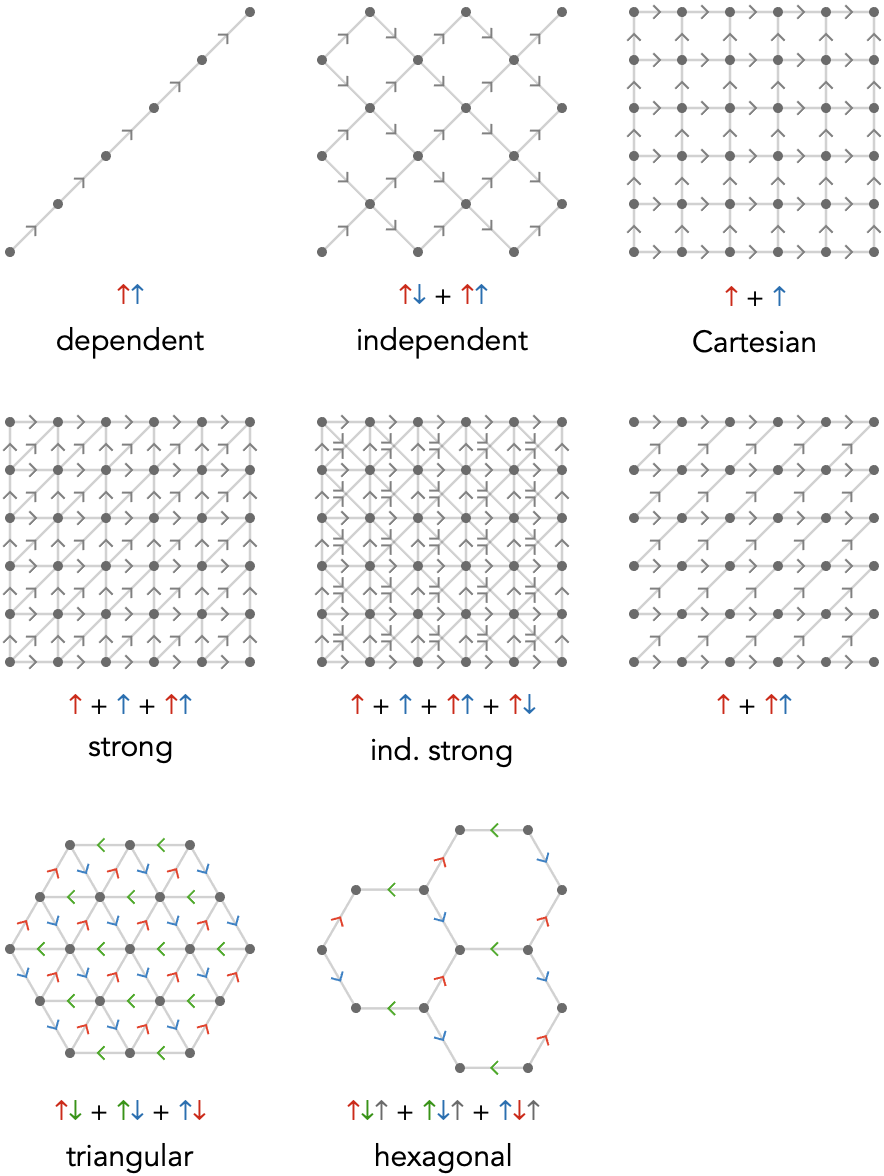In Path calculus, we define vertex and edge fields and use them to set up the machinery of finite differences on quivers.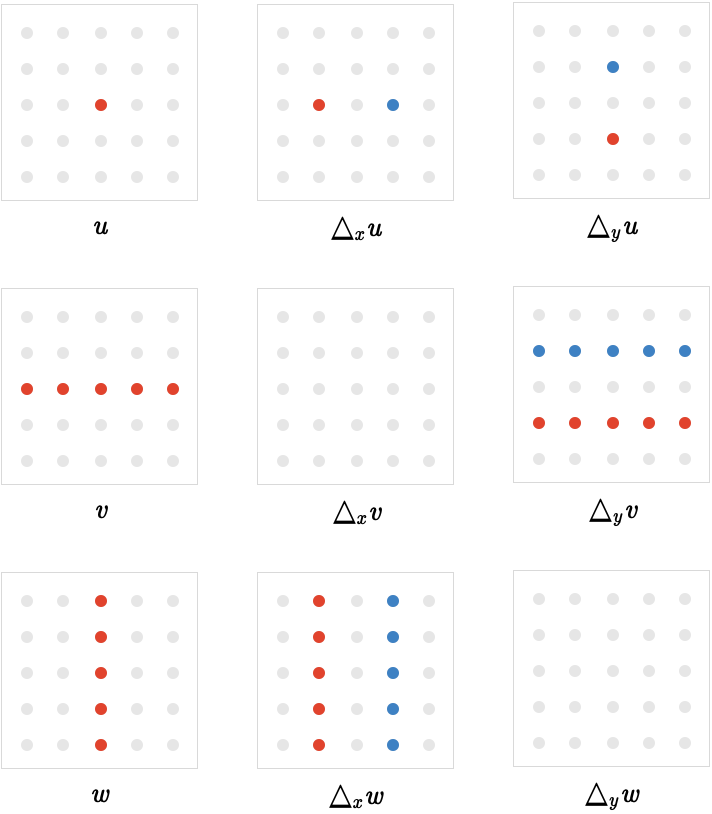In Path algebra, we unify vertex and edge fields into path vectors, and define the operations of translation, composition, and covariant difference.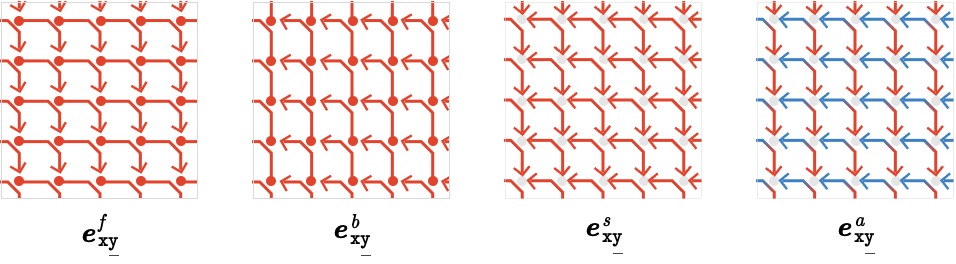In Cardinal transport, we create a Möbius quiver, equip it with charts, and use it to define a transport atlas that tracks transitions between parallel cardinals, giving us a method for cardinal transport. A suitable quotient yields the curvature quiver.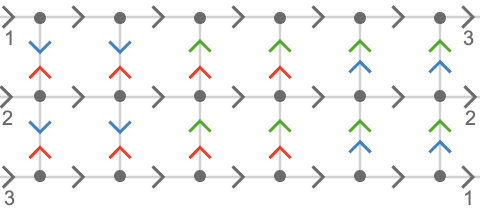In The cube, we set up an atlas on a quiver representing the Platonic cube, and calculate the transport atlas and curvature quiver, obtaining the Cayley quiver of the binary tetrahedral group.